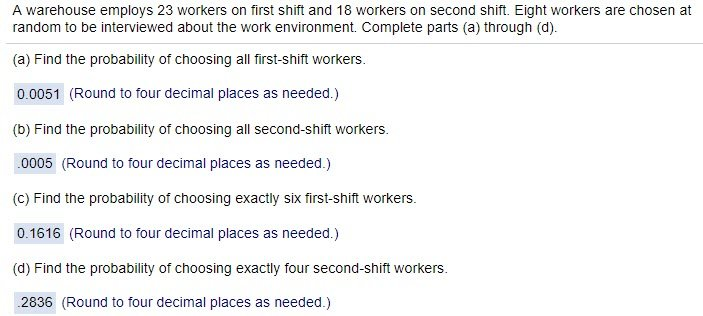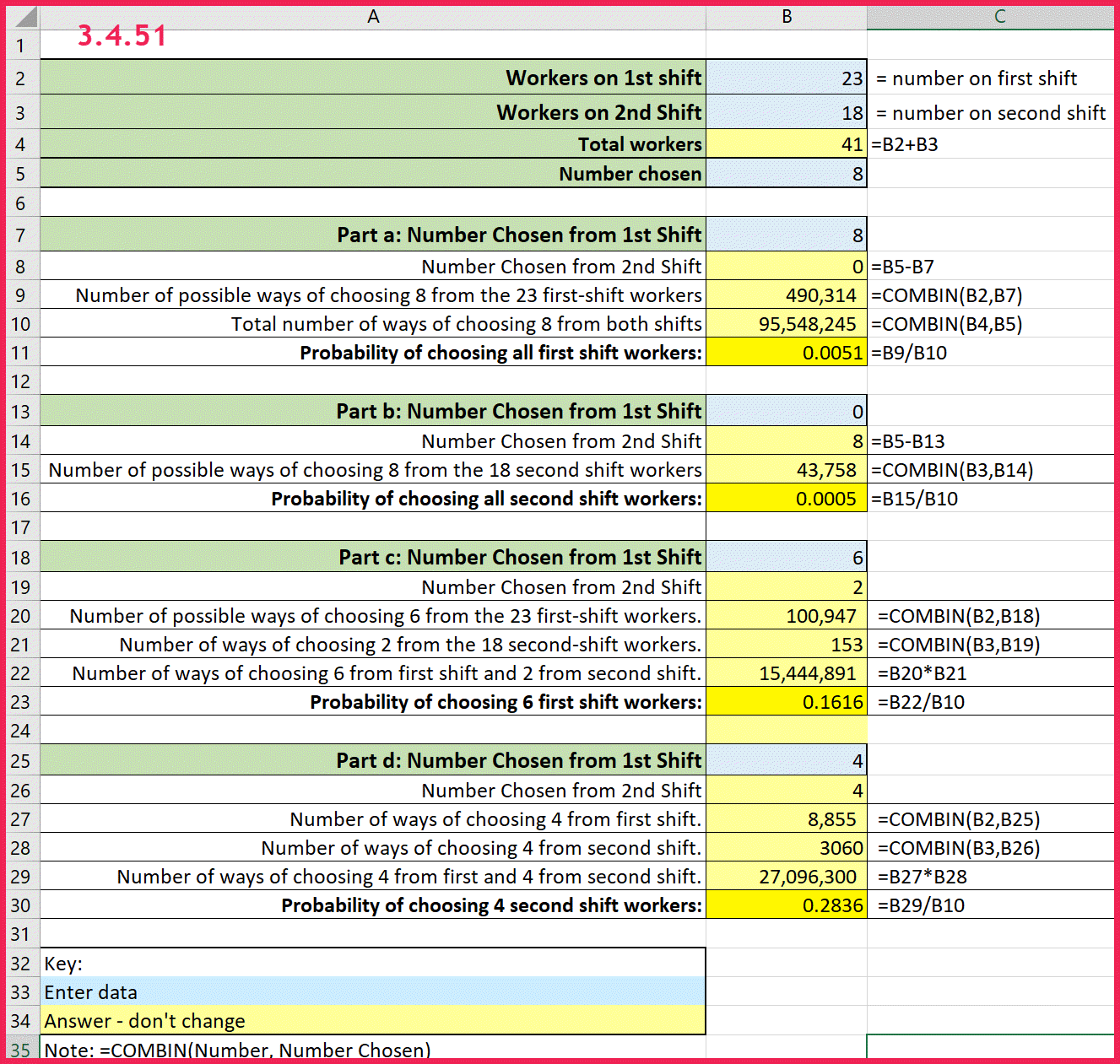# Combinations using basic Excel

I have a video here on solving combination and permutations problems using StatCrunch, but here is the basic Excel solution for problem 3.4.51 in MyStatLab homework which just requires the use of combinations since order does not matter.

Remember the fundamental counting principle says that if Event A can occur in m ways and Event B can occur in n ways, the number of ways Event A and Event B can occur is m*n.